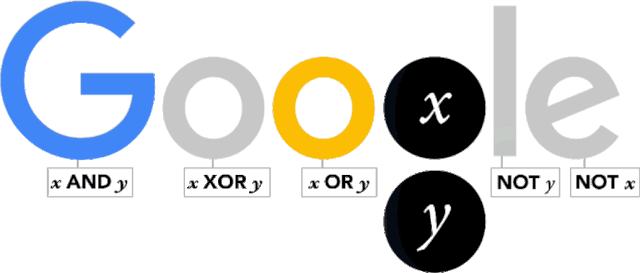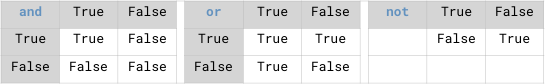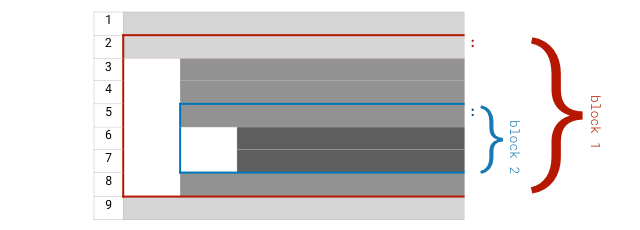Article Outline

# 入口

“速成”，对绝大多数人3来说，在绝大多数情况下，是不大可能的。

## 乔治・布尔

1833 年，一个 18 岁的英国小伙脑子里闪过一个念头：

1847 年，乔治 32 岁，出版了他人生的第一本书籍，THE MATHEMATICAL ANALYSIS OF LOGIC —— 18 岁那年的闪念终于成型。这本书很短，只有 86 页，但最终它竟然成了人类的瑰宝。在书里，乔治・布尔很好地解释了如何使用代数形式表达逻辑思想。

1849 年，乔治・布尔 34 岁，被当年刚刚成立的女皇学院（Queen's College）聘请为第一位数学教授。随后他开始写那本最著名的书，AN INVESTIGATION OF THE LAWS OF THOUGHT。他在前言里写到：

“The design of the following treatise is to investigate the fundamental laws of those operations of the mind by which reasoning is performed; to give expression to them in the symbolical language of a Calculus, and upon this foundation to establish the science of Logic and construct its method; …”

“本书论述的是，探索心智推理的基本规律；用微积分的符号语言进行表达，并在此基础上建立逻辑和构建方法的科学……”2015 年，乔治・布尔诞辰 200 周年，Google 设计了专门的 Logo 纪念这位为人类作出巨大贡献的自学奇才。A very happy 11001000th birthday to genius George Boole!

## 布尔运算

### 布尔值

NOTE

``````print(1 == 2)
print(1 != 2)``````
``````False
True``````

`1 == 2`，用自然语言描述就是 “1 等于 2 吗？” —— 它的布尔值当然是 `False`

`1 != 2`，用自然语言描述就是 “1 不等于 2 吗？” —— 它的布尔值当然是 `True`

NOTE

NOTE

### 逻辑操作符

Python 语言中的逻辑操作符（Logical Operators）如下表所示 —— 为了理解方便，也可以将其称为 “比较操作符”。

`==` 等于 `1 == 2` `False`
`!=` 不等于 `1 != 2` `True`
`>` 大于 `1 > 2` `False`
`>=` 大于等于 `1 >= 1` `True`
`<` 小于 `1 < 2` `True`
`<=` 小于等于 `1 <= 2` `True`
`in` 属于 `'a' in 'basic'` `True`

`'a'` 存在于 `'basic'` 这个字符串之中吗？”（属于关系）

### 布尔运算操作符

NOTE``````print('(True and False) yields:',  True and False)
print('(True and True) yields:',  True and True)
print('(False and True) yields:',  False and True)
print('(True or False) yields:',  True or False)
print('(False or True) yields:',  False or True)
print('(False or False) yields:',  False or False)
print('(not True) yields:',  not True)
print('(not False) yields:',  not False)``````
``````(True and False) yields: False
(True and True) yields: True
(False and True) yields: False
(True or False) yields: True
(False or True) yields: True
(False or False) yields: False
(not True) yields: False
(not False) yields: True``````## 流程控制

``````import random
r = random.randrange(1, 1000)
# 请暂时忽略以上两句的原理，只需要了解其结果：
# 引入随机数，而后，每次执行的时候，r 的值不同

if r % 2 == 0:
print(r, 'is even.')
else:
print(r, 'is odd.')``````
``693 is odd.``

``````    ...
if r % 2 == 0:
...
else:
...``````

`r = 2` 之中，`r` 是一个名称为 `r`变量（Variable）—— 现在只需要将变量理解为程序保存数值的地方；而 `=` 是赋值符号，`2` 是一个整数常量（Literal）。

“把 `2` 这个值保存到名称为 `r` 的变量之中”。

``````for i in range(10):
if i % 2 != 0:
print(i)``````
``````1
3
5
7
9``````

``````for i in range(10): # 对于 0～9 中的所有数字都带入 i 这个变量，执行一遍以下任务：
if i % 2 != 0:  #     如果 i 除以 2 的余数不等于零的话，执行下面的语句：
print(i)    #         向屏幕输出 i 这个变量中所保存的值``````

• `n` 为整数，`n >= 2`
• `n == 2``n` 是质数；
• `n > 2`，就把 `n` 作为被除数，从 `2` 开始一直到 `n - 1` 都作为除数，逐一计算看看余数是否等于 `0`
• 如果是，那就不用接着算了，它不是质数；
• 如果全部都试过了，余数都不是 `0`，那么它是质数。

``````for n in range(2, 100): #range(2,100)表示含左侧 2，不含右侧 100，是不是第三次看到这个说法了？
if n == 2:
print(n)
continue
for i in range(2, n):
if (n % i) == 0:
break
else:                  # 这里目前你可能看不懂…… 但先关注结果吧。
print(n)``````
``````2
3
5
7
11
13
17
19
23
29
31
37
41
43
47
53
59
61
67
71
73
79
83
89
97``````

## 所谓算法

`2` 作为除数开始试，试到 n 的 1/2 次方之后的一个整数就可以了……

``````for n in range(2, 100):
if n == 2:
print(n)
continue
for i in range(2, int(n ** 0.5)+1): #为什么要 +1 以后再说…… n 的 1/2 次方，相当于根号 n。
if (n % i) == 0:
break
else:
print(n)``````
``````2
3
5
7
11
13
17
19
23
29
31
37
41
43
47
53
59
61
67
71
73
79
83
89
97``````

## 所谓函数

``````a = abs(-3.1415926)
print(a)``````
``3.1415926``

• 我们调用了一个函数名`abs` 的函数；写法是 `abs(-3.1415926)`
• 这么写，就相当于向它传递了一个参数，其值为：`-3.1415926`
• 该函数接收到这个参数之后，根据这个参数的在函数内部进行了运算
• 而后该函数返回了一个值，返回值为之前接收到的参数的值的绝对值 `3.1415926`
• 而后这个被保存到变量 `a` 之中。

• 它有输入 —— 即，它能接收外部通过参数传递的值；
• 它有处理 —— 即，内部有能够完成某一特定任务的代码；尤其是，它可以根据 “输入” 得到 “输出”；
• 它有输出 —— 即，它能向外部输送返回值……

``````def is_prime(n):            # 定义 is_prime()，接收一个参数
if n < 2:              # 开始使用接收到的那个参数（值）开始计算……
return False       # 不再是返回给人，而是返回给调用它的代码……
if n == 2:
return True
for m in range(2, int(n**0.5)+1):
if (n % m) == 0:
return False
else:
return True

for i in range(80, 110):
if is_prime(i):          # 调用 is_prime() 函数，
print(i)            # 如果返回值为 True，则向屏幕输出 i``````
``````83
89
97
101
103
107
109``````

## 细节补充

### 语句

``````for i in range(10):
if i % 2 != 0:
print(i)``````
``````1
3
5
7
9``````

### 语句块NOTE

### 赋值符号与操作符的连用

`x = x + 1` 的意思是说，把表达式 `x + 1` 的值保存到变量 `x` 中去 —— 如此这般之后，`x` 这个变量中所保存的就不再是原来的值了……

``````x = 0
x += 1
print(x)``````
``1``

``````x = 11
x %= 3   # x = x % 3
print(x)``````
``2``

## 总结

• 数据：整数、布尔值；操作符；变量、赋值；表达式
• 函数、子程序、参数、返回值、调用
• 流程控制、分支、循环
• 算法、优化
• 程序：语句、注释、语句块
• 输入、处理、输出
• 解释器

1. ^ 对于自学能力强、有很多自学经验的人来说，速成往往真的是可能、可行的。因为他们已经积累的知识与经验会在习得新技能时发挥巨大的作用，乃至于他们看起来相对别人花极少的时间就能完成整个自学任务。也就是说，将来的那个已经习得自学能力、且自学能力已经磨练得很强的你，常常真的可以做到在别人眼里 “速成”。